O-minimal

(diff) ← Older revision | Latest revision (diff) | Newer revision → (diff)

Letbe a first-order language containing a binary relation symbol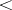and letbe an-structure (cf. Structure) in whichis interpreted as a total order (cf. Order (on a set)). Thenis called-minimal if every parametrically definable subset ofis a finite union of intervals of. An interval ofis a subset of the formfor some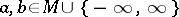, where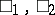stand foror. For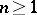, a subsetof the Cartesian productis called parametrically definable if there are an-formula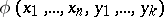and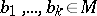such that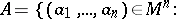An elementary theory is called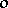-minimal if every model of it is-minimal.

This notion was introduced by L. van den Dries in [a2], while studying the expansionof the ordered fieldof the real numbers by the real exponential function (cf. Exponential function, real). He observed that the sets parametrically definable in Cartesian products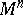for an-minimal expansionofshare many of the geometric properties of semi-algebraic sets (cf. Semi-algebraic set). For example, a semi-algebraic set has only finitely many connected components, each of them semi-algebraic (cf. [a1]), and van den Dries showed that this result remains true if one replaces "semi-algebraic" by "parametrically definable in an o-minimal expansion of R" . This is a finiteness theorem, and van den Dries aims to explain the other finiteness phenomena in real algebraic and real analytic geometry as consequences of-minimality (cf. [a3]).

In [a6], J.F. Knight, A. Pillay and C. Steinhorn prove the following results.

1)-minimality is preserved under elementary equivalence.

2) An ordered group is-minimal if and only if it is divisible Abelian.

3) An ordered ring is-minimal if and only if it is a real closed field.

4) Any parametrically definable unary function in an-minimal structure is piecewise strictly monotone or constant, and continuous. The real closed fieldis-minimal. The expansion ofby restricted analytic functions (cf. Model theory of the real exponential function) is-minimal (cf. [a4]), as a consequence of Gabrielov's theorem of the complement that the complement of a subanalytic set is subanalytic (cf. [a5]). It follows from work of A. Wilkie [a7] thatis-minimal. His recent generalization of Gabrielov's theorem establishes the much stronger result that the expension ofby Pfaffian chains of total functions is-minimal, see [a8]. A. Macintyre, van den Dries and D. Marker establish in [a4] the-minimality ofexpanded by the restricted analytic functions and the exponential function. For a research account on-minimal structures, see [a3].

How to Cite This Entry:
O-minimal. Encyclopedia of Mathematics. URL: http://encyclopediaofmath.org/index.php?title=O-minimal&oldid=12517
This article was adapted from an original article by S. Kuhlmann (originator), which appeared in Encyclopedia of Mathematics - ISBN 1402006098. See original article